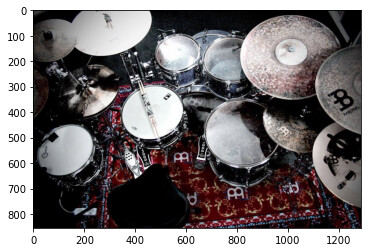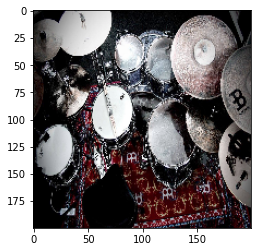# How to do image resizing with bilinear interpolation using torch tensor on CUDA?

Hi all,

I was wondering whether has anyone done bilinear interpolation resizing with PyTorch Tensor under CUDA?

I tried this using `torch.nn.functional.F.interpolate(rgb_image,(size,size))` and it works to resize the RGB image tensor shape (batch,channel,size,size). However, the default mode is ‘nearest’ and when I change to ‘bilinear’, it comes with an error about the tensor type which I fixed by changing to `torch.FloatTensor` but then the output shown is grainy and pixelated with noise. My command:

``````resized = F.interpolate(rgb_image_tensor.type(torch.FloatTensor),(256,256),mode='bilinear')
``````

I show the image by using

``````result =  torchvision.transform.functional.to_pil_image(resized)
result.show()
``````

Default way works, shows the image is resized accordingly but ‘bilinear’ shows noisy image, any clues?

‘bilinear’ mode output:Your output might be clipped to `[0, 1]`, if you are trying to visualize floating point numbers or `[0, 255]` if you are using `uint8`.
I cannot reproduce the issue using the latest stable releases and by making sure I’m casting to the expected type:

``````img = PIL.Image.open('drums.png')
img_arr = np.array(img)[:, :, :3] # remove alpha channel
plt.imshow(img_arr)

x = torch.from_numpy(img_arr).permute(2, 0, 1).unsqueeze(0).float()

out1 = F.interpolate(x, size=(200, 200))
out2 = F.interpolate(x, size=(200, 200), mode='bilinear')

plt.imshow(out1.permute(1, 2, 0).byte().numpy())
plt.imshow(out2.permute(1, 2, 0).byte().numpy())
``````

Original`out1``out2`1 Like

You are right! Thank you for your help, appreciate it!

I just needed to cast the bilinear output as `.byte()`! I did not realised this as `mode='nearest'` is able to take in tensor as byte type and output given is already in byte. Meanwhile, `mode='bilinear` needs FloatTensor and outputs in float. Thus, to show the image, we have to put it back to byte type.

I am actually amazed that pytorch has implemented resizing for GPU. Now I can skip using `cv2.resize()` and remain the tensor on the GPU for resizing instead!

Any idea on whether are there major differences between `cv2.resize() -> bilinear` to `F.interpolate(....,mode='bilinear')`? Your comments are appreciated.

I haven’t checked the latest updates in torchvision, but know that there was at least work in progress to avoid small numerical differences between different cv libraries.
You could run a quick test with the latest `torchvision` release (`0.10.0`) or the nightly and see how large these differences would be.

thank you for the help and reply.

Actually, I realised that it matters more that the `torchvision.transform.resize()` is same as `torch.nn.functional.interpolate()` for my use case as the model is trained and tested under torchvision transformation for the DataLoader.

Just to complete this thread for anyone interested, I found that both functions is the same:

`torchvision.transform.resize()` calls to this `resize()` function here vision/functional_tensor.py at ab60e538961e4bb25e7d8db44a4d0d96155e3644 · pytorch/vision · GitHub which imports `interpolate` from `torch.nn.functional`